# Lorenz Attractor (Butterfly Effect)The Lorenz attractor is based on a set of three equations (called the Lorenz equations) that describes the pseudo chaotic trajectory of a point in the 3D space. These equations have been found by Edward Norton Lorenz when he worked on complex weather simulations at the MIT.

The three equations are the following:

```dx/dt = S (y - x)
dy/dt = x (R - z) - y
dz/dt = xy - Bz
```

where S, R and B are the system parameters (constants). These equations allow to compute the variation of the position for a small variation of time.

We get this well-known picture for a particular value of the three parameters (S=10, R=28 and B=8/3):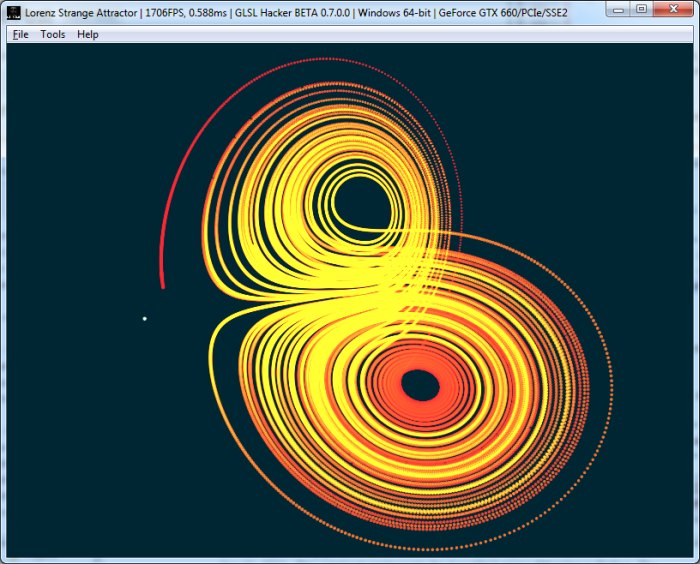Lorenz attractor for S=10, R=28 and B=8/3

But other values produce similar shapes like {S=10, R=30, B=4} or {S=45, R=45, B=8/3}, see screenshots at the end of the article.

The Butterfly Effect: two initial positions with a very small difference (for example P0={x=2; y=2.02; z=2} and P1={x=2.01; y=2.03; z=2.01}) will produce two different trajectories. In the following screenshots, the difference between the initial positions is 0.01: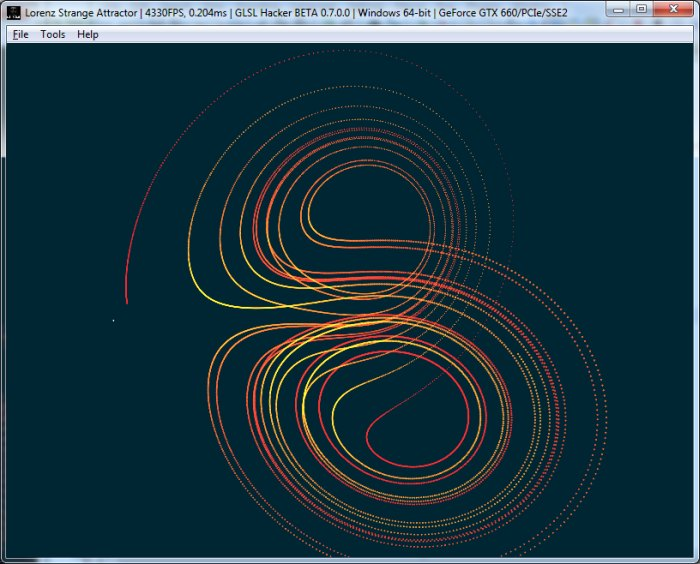butterfly effect – P0={x=2; y=2.02; z=2} – S=10, R=28 and B=8/3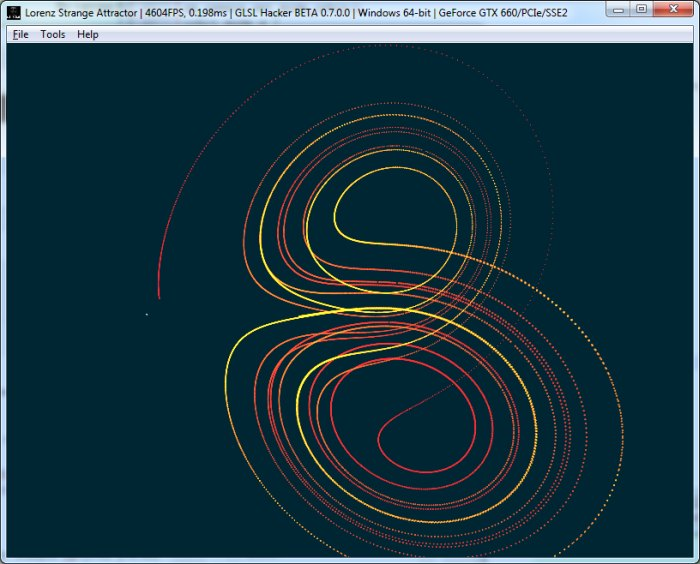butterfly effect – P0={x=2.01; y=2.03; z=2.01} – S=10, R=28 and B=8/3

The name butterfly effect comes from the shape of the trajectory that looks like the wings of a butterfly. Here is an explanation of the bufferfly effect from wikipedia:

In chaos theory, the butterfly effect is the sensitive dependency on initial conditions in which a small change at one place in a deterministic nonlinear system can result in large differences in a later state. The name of the effect, coined by Edward Lorenz, is derived from the theoretical example of the details of a hurricane (exact time of formation, exact path taken) being influenced by minor perturbations equating to the flapping of the wings of a distant butterfly several weeks earlier. Lorenz discovered the effect when he observed that runs of his weather model with initial condition data that was rounded in a seemingly inconsequential manner would fail to reproduce the results of runs with the unrounded initial condition data. A very small change in initial conditions had created a significantly different outcome.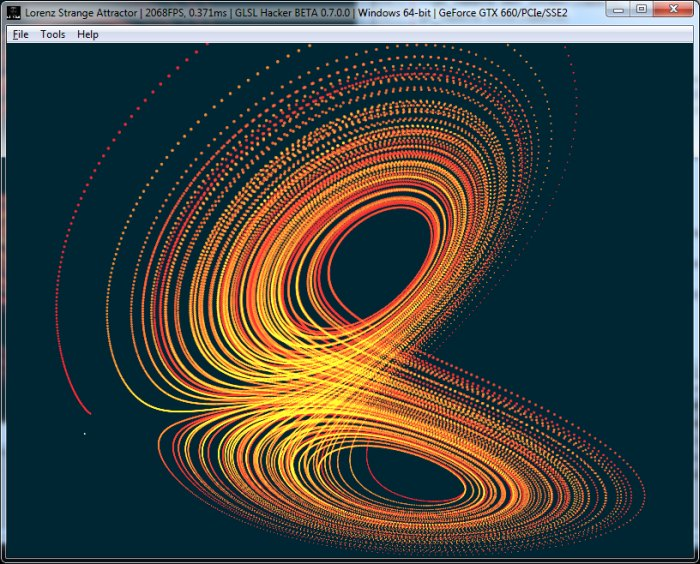The wings of a butterfly

Here is a code snippet that shows how to plot a point P:

```function InitPosition()
P.x = random()
P.y = random()
P.z = random()
end

function UpdatePosition(dt)
dx = 10 * (P.y - P.x) * dt
dy = (P.x * (28 - P.z) - P.y) * dt
dz = (P.x * P.y - 8/3 * P.z) * dt

P.x = P.x + dx
P.y = P.y + dy
P.z = P.z + dz
end

InitPosition()
while(true)
UpdatePosition(dt)
DrawPoint(P)
end
```

I coded a small demo with GLSL Hacker that visualizes the path of a point following Lorenz equations. And to make the demo more compelling, each and every position is displayed, showing the full trajectory instead of a single point that moves from one position to the other one.

The demo (in Lua + GLSL) is available in the host_api/Particle_Lorenz_Attractor/ folder of GLSL Hacker demopack. The demo uses a vertex pool (an big array of vertices) to render the Lorenz attractor. To set the initial position, look at around line 81. To change the Lorenz equations parameters (S, R and B), jump to line 180.

Here are some shapes of the Lorenz attractor for various values of S, R and B: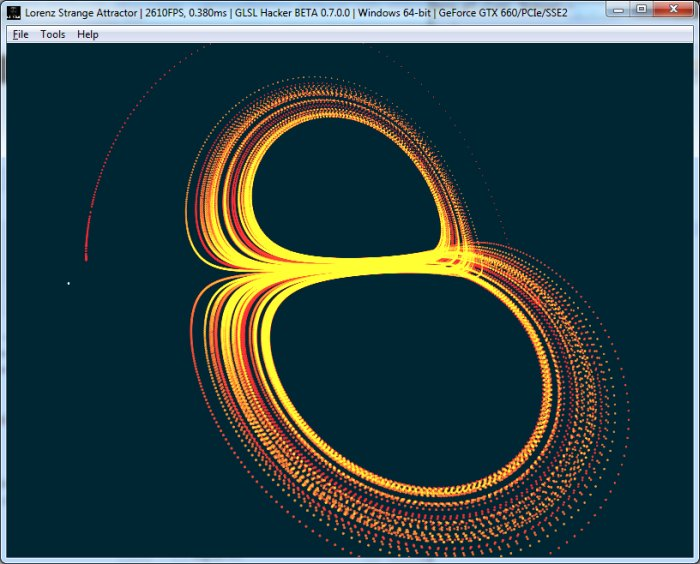Lorenz attractor for S=45, R=45 and B=8/3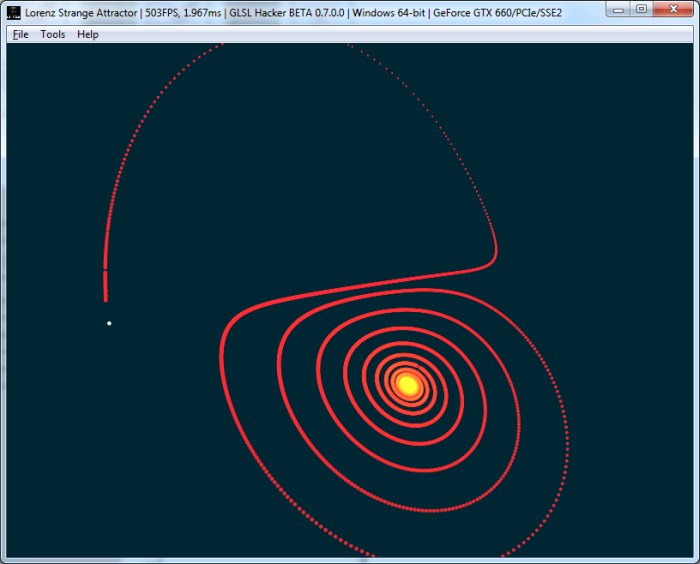Lorenz attractor for S=60, R=28 and B=8/3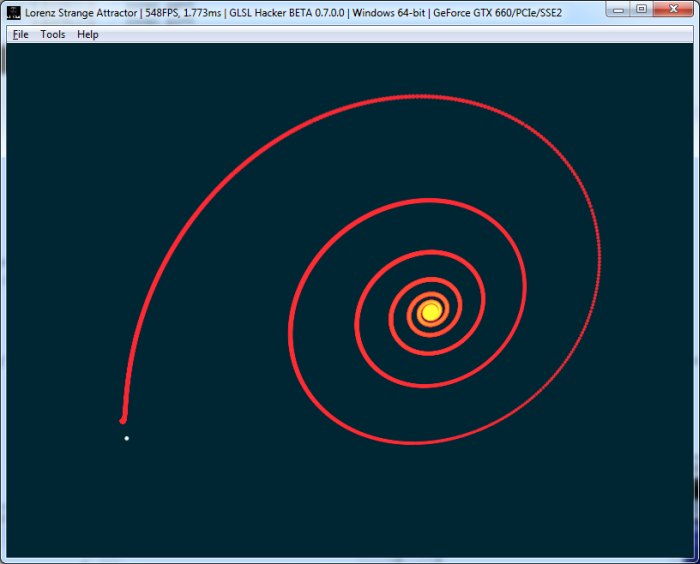Lorenz attractor for S=2, R=28 and B=8/3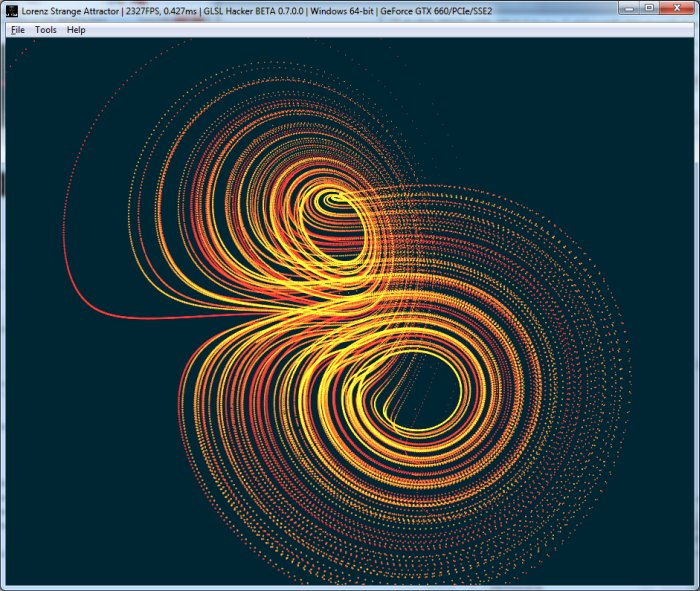Lorenz attractor for S=10, R=60 and B=8/3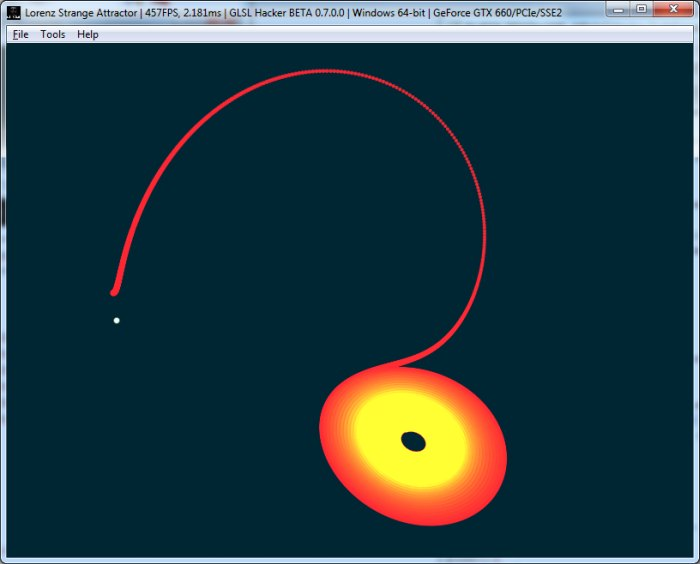Lorenz attractor for S=10, R=20 and B=8/3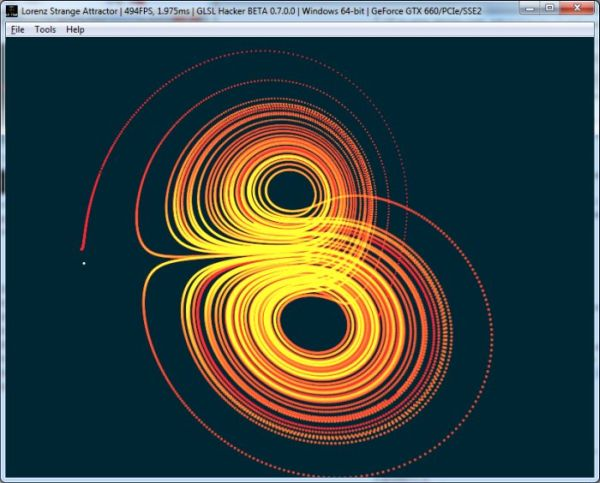Lorenz attractor for S=12, R=30 and B=2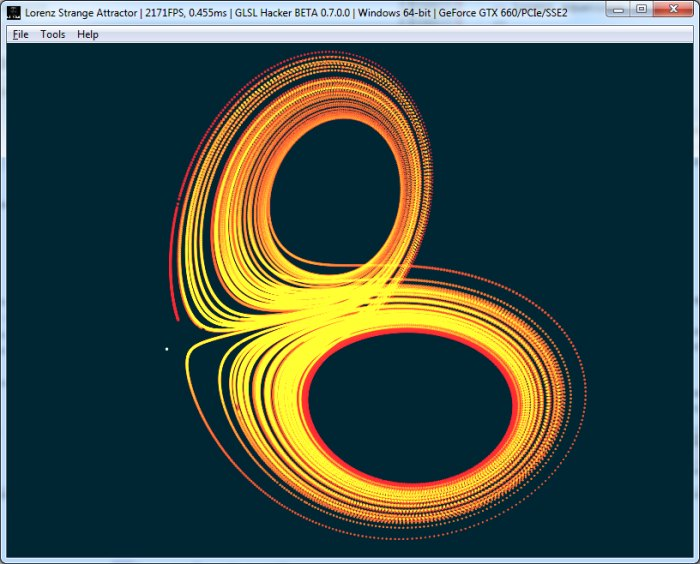Lorenz attractor for S=10, R=30 and B=4.5